# 11. Input devices¶

I planned to build a circuit to read from an ultrasonic range sensor, to measure distance in centimeters and divide the distances into 3 regions.

1. Close -> Gives Red Light
2. Near -> Gives Yellow Light
3. Far -> Gives Green Light

so basically we are goin to add the sensor to the previous example which have 3 traffic light LEDs.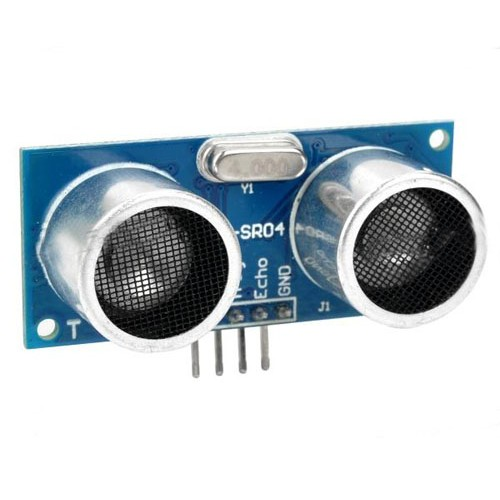this sensor works like a radar through detection of doppler effect which means its sends a signal through the air and starts a timer and monitors the receiver for any echo coming back, once the echo is detected the timer stops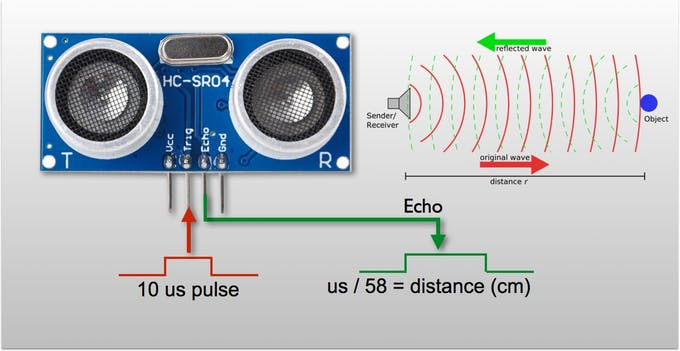to know the exact distance we refer to the physics low of speed

``````speed = distance / time
``````

here in this equation speed of sound in air is know with a constant 343 meters per second, (time) its the time taken from sending out the signal until echo is detected in seconds, but because the sound traveled back and forth that means the sound traveled twice the distance therefore should be divided by two

``````speed of sound = distance / (2 x time)
``````

then the distance can be calculated

``````distance = speed of sound x (2 x time)
``````

how ever this is just and explanation but the library will do it for use all we need to do is download the library and include it then use it to measure distances. the sensor wiring is as the following

To Download the library GoTo -> Sketch -> Include Library -> Manage Libraries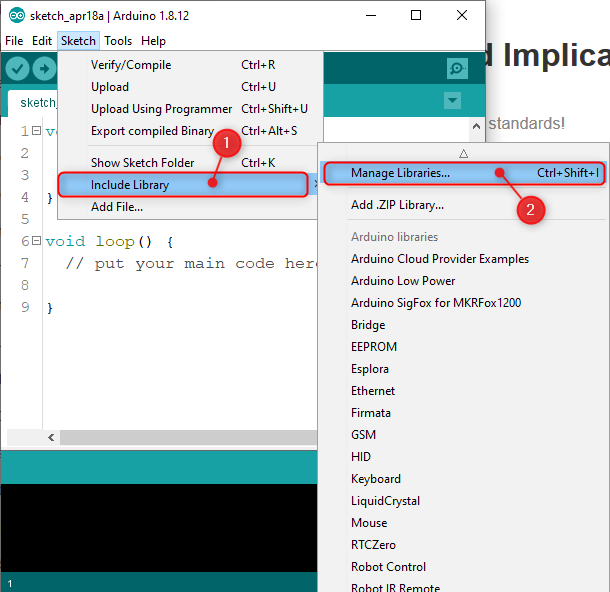In The Text Box Type Ultrasonic and then install the library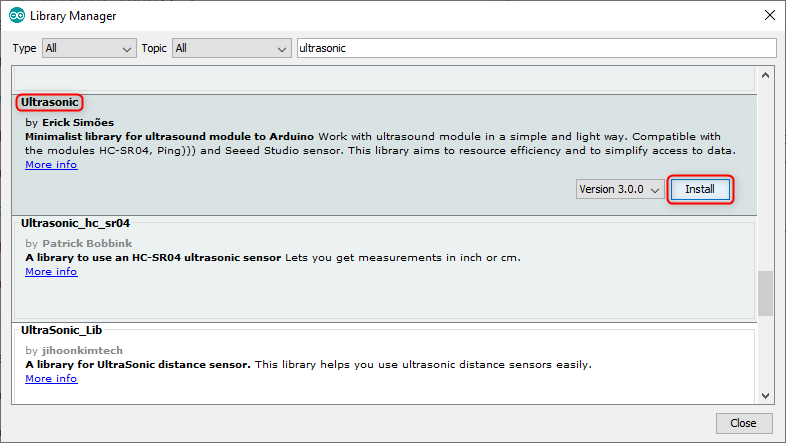Wiring the ultrasonic module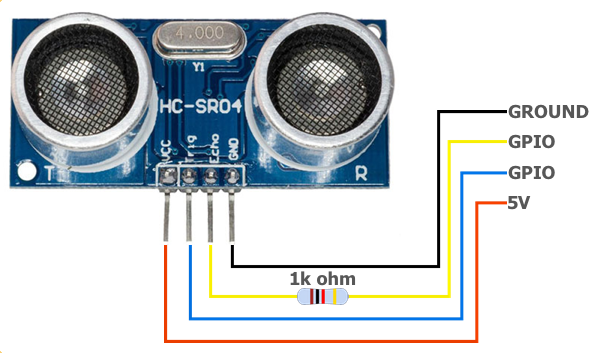• Ground Black Wire -> GND on Arduino
• GPIO Blue Wire (Trigger) -> Pin Number 9 On Arduino
• GPIO Yellow Wire (Echo) -> Pin Number 8 On Arduino
• 5V Red Wire -> +5V Pin On Arduino
###### Code¶
``````// Include Libraries
#include <Arduino.h>
#include <Ultrasonic.h>

// Define Pins
#define   RED     2
#define   YELLOW  3
#define   GREEN   4

// Make An Object Name Ultrasonic To Read Distance
Ultrasonic ultrasonic(9,8);

void setup() {
Serial.begin(9600);
pinMode(RED, OUTPUT);
pinMode(YELLOW, OUTPUT);
pinMode(GREEN, OUTPUT);
}

void loop() {

// This Will Read The Current Distance
float distance = ultrasonic.Ranging(CM);

// This Will Print For Us The Distance In Centimeters
Serial.println(String(distance, 2) + " cm");

if(distance > 0 and distance < 5) {

// This Means That The Object Is Too Close
digitalWrite(RED, HIGH);
digitalWrite(YELLOW, LOW);
digitalWrite(GREEN, LOW);

} else if(distance > 5 and distance < 10) {

// This Means That The Object Is Medium Distance
digitalWrite(RED, LOW);
digitalWrite(YELLOW, HIGH);
digitalWrite(GREEN, LOW);

} else if(distance > 10 and distance < 20) {
// This Means That The Object Is Too Far
digitalWrite(RED, LOW);
digitalWrite(YELLOW, LOW);
digitalWrite(GREEN, HIGH);
}

// Delay of 1/4 Second
delay(250);
}
``````